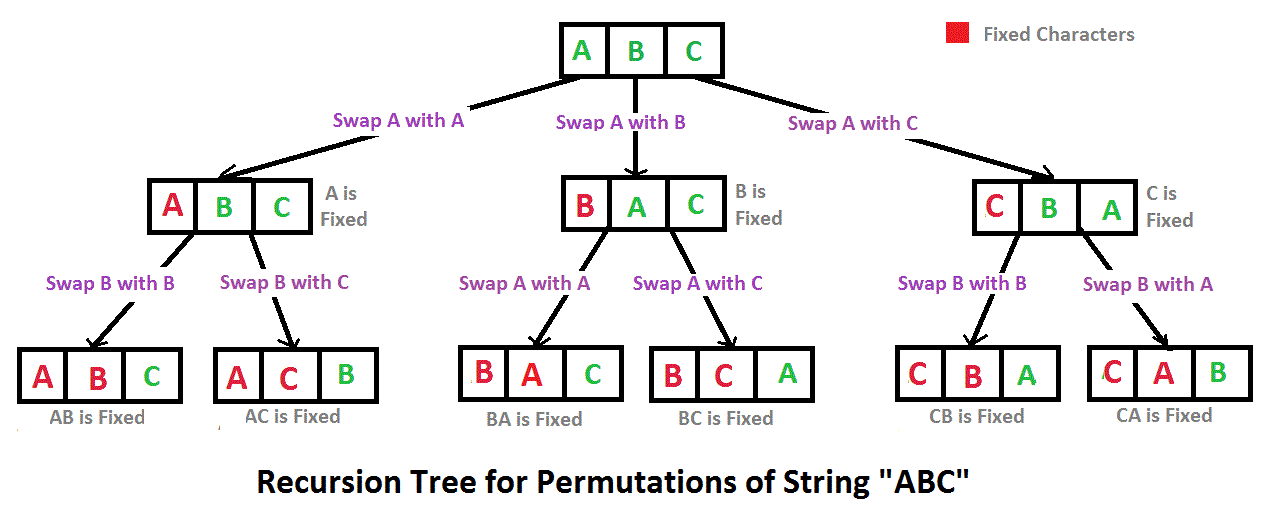# Python Program to print all permutations of a given string

• Difficulty Level : Medium
• Last Updated : 13 Jan, 2022

A permutation also called an “arrangement number” or “order,” is a rearrangement of the elements of an ordered list S into a one-to-one correspondence with S itself. A string of length n has n! permutation.

Source: Mathword(http://mathworld.wolfram.com/Permutation.html)

Below are the permutations of string ABC.
ABC ACB BAC BCA CBA CAB

Here is a solution that is used as a basis in backtracking.## Python3

 `# Python program to print all permutations``# with duplicates allowed``def` `toString(``List``):``    ``return` `''.join(``List``)` `# Function to print permutations``# of string``# This function takes three parameters:``# 1. String``# 2. Starting index of the string``# 3. Ending index of the string.``def` `permute(a, l, r):``    ``if` `l ``=``=` `r:``        ``print` `(toString(a))``    ``else``:``        ``for` `i ``in` `range``(l, r ``+` `1``):``            ``a[l], a[i] ``=` `a[i], a[l]``            ``permute(a, l ``+` `1``, r)` `            ``# backtrack``            ``a[l], a[i] ``=` `a[i], a[l]` `# Driver code``string ``=` `"ABC"``n ``=` `len``(string)``a ``=` `list``(string)``permute(a, ``0``, n``-``1``)``# This code is contributed by Bhavya Jain`

Output:

```ABC
ACB
BAC
BCA
CBA
CAB```

Time Complexity: O(n*n!) Note that there are n! permutations and it requires O(n) time to print a permutation.

Auxiliary Space: O(r – l)

Note: The above solution prints duplicate permutations if there are repeating characters in the input string. Please see the below link for a solution that prints only distinct permutations even if there are duplicates in input.
Print all distinct permutations of a given string with duplicates.
Permutations of a given string using STL

Another approach:

## Python3

 `# Python program to implement``# the above approach``def` `permute(s, answer):``    ``if` `(``len``(s) ``=``=` `0``):``        ``print``(answer, end ``=` `"  "``)``        ``return``    ` `    ``for` `i ``in` `range``(``len``(s)):``        ``ch ``=` `s[i]``        ``left_substr ``=` `s[``0``:i]``        ``right_substr ``=` `s[i ``+` `1``:]``        ``rest ``=` `left_substr ``+` `right_substr``        ``permute(rest, answer ``+` `ch)` `# Driver Code``answer ``=` `""``s ``=` `input``(``"Enter the string : "``)``print``(``"All possible strings are : "``)``permute(s, answer)``# This code is contributed by Harshit Srivastava`

Output:

```Enter the string : abc
All possible strings are : abc  acb  bac  bca  cab  cba```

Time Complexity: O(n*n!) The time complexity is the same as the above approach, i.e. there are n! permutations and it requires O(n) time to print a permutation.

Auxiliary Space: O(|s|)

My Personal Notes arrow_drop_up